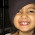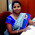## Sunday, March 11, 2012

### IMPLEMENTATION OF DISK SCHEDULING ALGORITHMS

 Ex. No. :11 IMPLEMENTATION OF DISK SCHEDULING ALGORITHMS

AIM:
To write a ‘C’ program to implement the Disk Scheduling algorithm for First Come First Served (FCFS), Shortest Seek Time First (SSTF), and SCAN.

PROBLEM DESCRIPTION:
Disk Scheduling is the process of deciding which of the cylinder request is in the ready queue is to be accessed next.
The access time and the bandwidth can be improved by scheduling the servicing of disk I/O requests in good order.
Access Time:
The access time has two major components:  Seek time and Rotational Latency.
Seek Time:
Seek time is the time for disk arm to move the heads to the cylinder containing the desired sector.
Rotational Latency:
Rotational latency is the additional time waiting for the disk to rotate the desired sector to the disk head.
Bandwidth:
The disk bandwidth is the total number of bytes transferred, divided by the total time between the first request for service and the completion of the last transfer.
ALGORITHM:
1. Input the maximum number of cylinders and work queue and its head starting position.
2.  First Come First Serve Scheduling (FCFS) algorithm – The operations are performed in order requested.
3.  There is no reordering of work queue.
4. Every request is serviced, so there is no starvation.
5. The seek time is calculated.
6. Shortest Seek Time First Scheduling (SSTF) algorithm – This algorithm selects the request with the minimum seek time from the current head position.
7. Since seek time increases with the number of cylinders traversed by the head, SSTF chooses the pending request closest to the current head position.
8. The seek time is calculated.
9. SCAN Scheduling algorithm – The disk arm starts at one end of the disk, and moves toward the other end, servicing requests as it reaches each cylinder, until it gets to the other end of the disk.
10. At the other end, the direction of head movement is reversed, and servicing continues.
11. The head continuously scans back and forth across the disk.
12. The seek time is calculated.
13. Display the seek time and terminate the program

Program :

#include<stdio.h>
#include<math.h>

void fcfs(int noq, int qu, int st)
{
int i,s=0;
for(i=0;i<noq;i++)
{
s=s+abs(st-qu[i]);
st=qu[i];
}
printf("\n Total seek time :%d",s);
}

void sstf(int noq, int qu, int st, int visit)
{
int min,s=0,p,i;
while(1)
{
min=999;
for(i=0;i<noq;i++)
if (visit[i] == 0)
{
if(min > abs(st - qu[i]))
{
min = abs(st-qu[i]);
p = i;
}
}
if(min == 999)
break;
visit[p]=1;
s=s + min;
st = qu[p];
}
printf("\n Total seek time is: %d",s);
}

void scan(int noq, int qu, int st, int ch)
{
int i,j,s=0;
for(i=0;i<noq;i++)
{
if(st < qu[i])
{
for(j=i-1; j>= 0;j--)
{
s=s+abs(st - qu[j]);
st = qu[j];
}
if(ch == 3)
{
s = s + abs(st - 0);
st = 0;
}
for(j = 1;j < noq;j++)
{
s= s + abs(st - qu[j]);
st = qu[j];
}
break;
}
}
printf("\n Total seek time : %d",s);
}

int main()
{
int n,qu,st,i,j,t,noq,ch,visit;
printf("\n Enter the maximum number of cylinders : ");
scanf("%d",&n);
printf("enter number of queue elements");
scanf("%d",&noq);
printf("\n Enter the work queue");
for(i=0;i<noq;i++)
{
scanf("%d",&qu[i]);
visit[i] = 0;
}
printf("\n Enter the disk head starting posision: \n");
scanf("%d",&st);
while(1)
{
printf("\n\n\t\t MENU \n");
printf("\n\n\t\t 1. FCFS \n");
printf("\n\n\t\t 2. SSTF \n");
printf("\n\n\t\t 3. SCAN \n");
printf("\n\n\t\t 4. EXIT \n");
printf("\nEnter your choice: ");
scanf("%d",&ch);
if(ch > 2)
{
for(i=0;i<noq;i++)
for(j=i+1;j<noq;j++)
if(qu[i]>qu[j])
{
t=qu[i];
qu[i] = qu[j];
qu[j] = t;
}
}
switch(ch)
{
case 1: printf("\n FCFS \n");
printf("\n*****\n");
fcfs(noq,qu,st);
break;

case 2: printf("\n SSTF \n");
printf("\n*****\n");
sstf(noq,qu,st,visit);
break;
case 3: printf("\n SCAN \n");
printf("\n*****\n");
scan(noq,qu,st,ch);
break;
case 4: exit(0);
}
}
}

Output

"disksche.c" 122L, 2076C written
[anandh@localhost ~]\$ cc disksche.c
[anandh@localhost ~]\$ ./a.out

Enter the maximum number of cylinders : 200
enter number of queue elements5

Enter the work queue23
89
132
42
187

Enter the disk head starting posision:
100

MENU

1. FCFS

2. SSTF

3. SCAN

4. EXIT

Enter your choice: 1

FCFS

*****

Total seek time : 421

MENU

1. FCFS

2. SSTF

3. SCAN

4. EXIT

Enter your choice: 2

SSTF

*****

Total seek time is: 273

MENU

1. FCFS

2. SSTF

3. SCAN

4. EXIT

Enter your choice: 3

SCAN

*****

Total seek time : 287

MENU

1. FCFS

2. SSTF

3. SCAN

4. EXIT

Enter your choice: 4

Result:
Thus the C program to implement the Disk scheduling algorithms namely FCFS, SSTF,
and SCAN algorithms was written and executed. The obtained outputs were verified.

#### 7 comments:

1.very helpfull.....tanx a lot.........

1.most welcome

2.how do the prog set up for a disk request file...?

3.4.thank u for the prog..

5.Thank you so much , Sir !!!!!

6.Can v write the same algorithm using linked list implementation in c? How